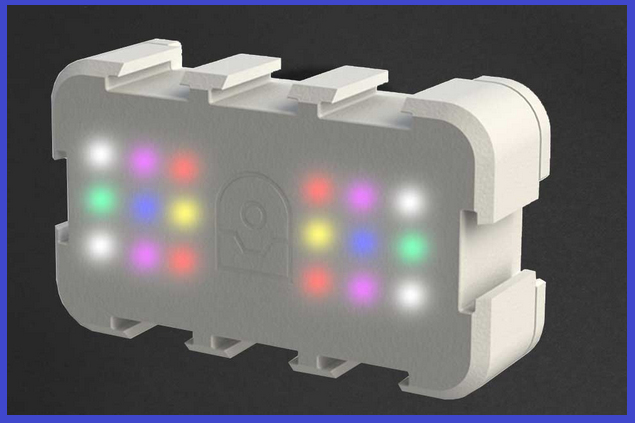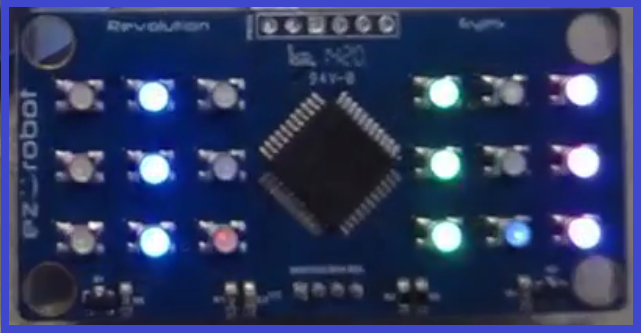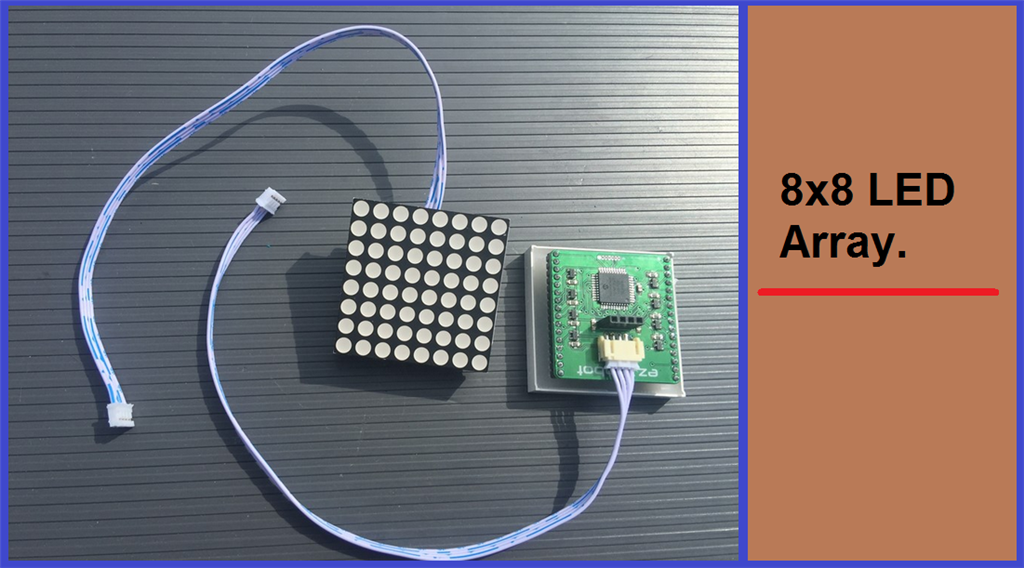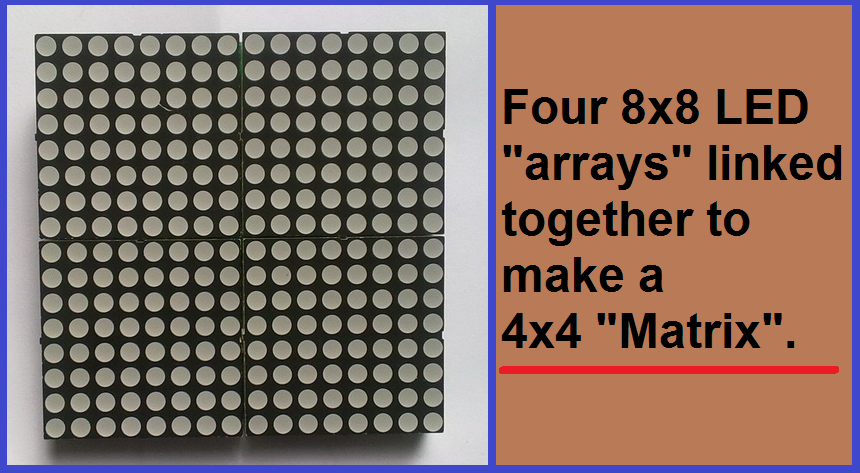## Explanation and Uses of Arrays.

##### Step 2. LED Array and Matrix

This step will give a short example of an LED array. An LED array is a series of LED's on a flat panel that form rows and columns. These can be made to display patterns or images (depending on how many LED's make up an array) either by using switching circuits and/or software like the RGB Animator found in EZ-Builder.With an RGB (red, green, blue) LED panel, each bulb (diode) has the same characteristics (they produce light and use the same power), but have different values (each RGB LED is made up of 3 different colour LED's), but as the characteristics and function are the same, it is still classed as an array. Now, if you were to chain multiple LED arrays together, this would form a matrix. In simple terms, a matrix is made up of multiple arrays. Let's look more closely at the RGB LED array seen above...The RGB LED Block is made up of 18 RGB LED's which adds up to 54 LED's in total (18 red, 18 green, 18 blue). The two sets of 3x3 RGB's you can see in the picture would make up a matrix, right? Not really, as both equal groups of bulbs are arranged in equal rows and on the same board which forms an array.

To use another type of LED LED array, below you will see on 8x8 LED array. Four of them chained together becomes an array of arrays. This is now a matrix.An array, is a fixed object or data structure.

A matrix, is made from multiple arrays. (Matrix has different meanings in different subjects such as maths, science and biology).

The next step will look at another hardware array.
RGB Animator